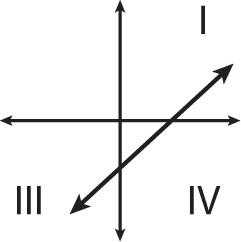# SAT Math Multiple Choice Question 457: Answer and Explanation

### Test Information

Question: 457

7. If the graph of y = mx + b passes through quadrants I, III, and IV on a coordinate plane, which of the following must be true about m and b?

• A. m < 0, b < 0
• B. m < 0, b > 0
• C. m > 0, b < 0
• D. m > 0, b > 0

Explanation:

C

Difficulty: Medium

Category: Heart of Algebra / Linear Equations

Strategic Advice: Draw a quick sketch of the line described and compare it to the answer choices. Don't forget, the quadrants start with I in the upper right corner and move counterclockwise.

Getting to the Answer: A sample sketch follows:Notice that the line is increasing, so the slope is positive (m > 0). This means you can eliminate A and B. The line crosses the y-axis below the origin, so b is negative (b < 0), which means (C) is correct.